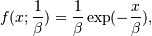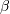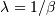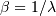# numpy.random.exponential¶

numpy.random.exponential(scale=1.0, size=None)

Draw samples from an exponential distribution.

Its probability density function isfor x > 0 and 0 elsewhere.is the scale parameter, which is the inverse of the rate parameter. The rate parameter is an alternative, widely used parameterization of the exponential distribution [R223].

The exponential distribution is a continuous analogue of the geometric distribution. It describes many common situations, such as the size of raindrops measured over many rainstorms [R221], or the time between page requests to Wikipedia [R222].

Parameters: scale : float or array_like of floats The scale parameter,. size : int or tuple of ints, optional Output shape. If the given shape is, e.g., (m, n, k), then m * n * k samples are drawn. If size is None (default), a single value is returned if scale is a scalar. Otherwise, np.array(scale).size samples are drawn. out : ndarray or scalar Drawn samples from the parameterized exponential distribution.

References

 [R221] (1, 2) Peyton Z. Peebles Jr., “Probability, Random Variables and Random Signal Principles”, 4th ed, 2001, p. 57.
 [R222] (1, 2) Wikipedia, “Poisson process”, http://en.wikipedia.org/wiki/Poisson_process
 [R223] (1, 2) Wikipedia, “Exponential distribution”, http://en.wikipedia.org/wiki/Exponential_distribution

#### Previous topic

numpy.random.dirichlet

numpy.random.f